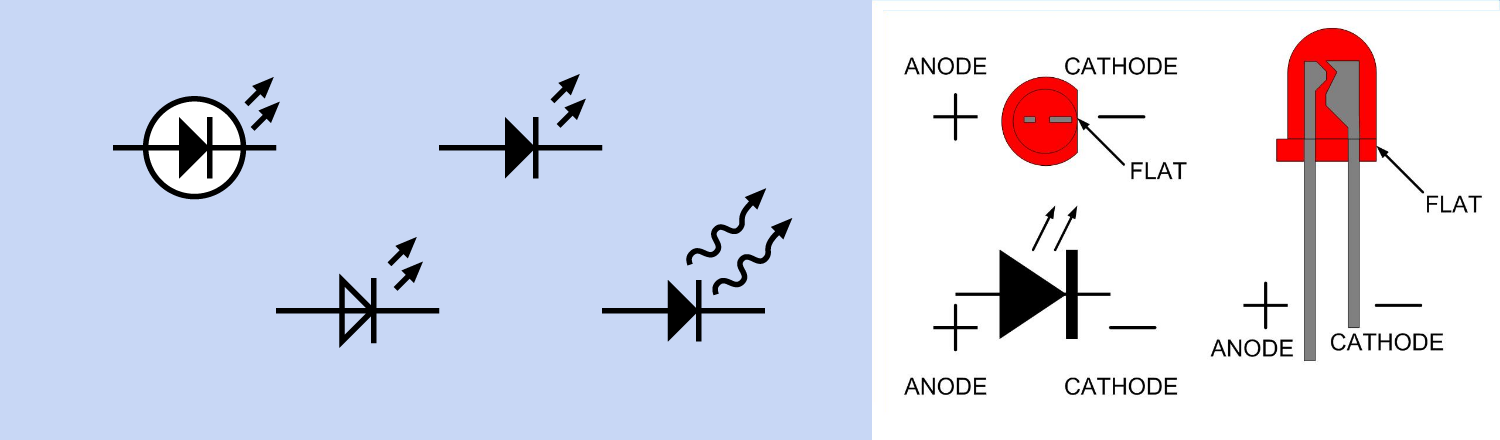Petter Holt Juliussen • Mail | GitHub | Letterboxd

# LEDs

2019-04-04

An LED indicator emits light in response to a small current, typically around 20 mA (but sometimes much less), at a voltage lower than 5VDC. An LED, like any diode, contains a semiconductor PN junction that conducts current only in the forward direction (i.e. from the more-positive side of a power supply to the more-negative side). The amount of energy released depends on the band gap, which is a property of the semiconductor material. The band gap also determines the threshold voltage of the LED. For this reason, LEDs of different colors have widely different threshold voltages.Figure 1: Various symbols may be used to represent an LED.

Because an LED will often be used in devices where the DC power supply exceeds the maximum forward voltage, a series resistor is customarily used as a simple way to restrict current through the diode. To eliminate the chore of adding a series resistor to limit current through an LED, some indicators are sold with a series resistor built in. They may be rated for use with 5 VDC or 12 VDC, but are externally indistinguishable from each other (and from LEDs that do not contain series resistors).

A through-hole LED will have two leads of unequal length. The longer lead connects internally with the anode of the diode, and should be wired externally to the “more positive” side of a power source. The shorter lead connects internally with the cathode of the diode, and should be wired externally to the “more negative” side of a power source.

## Resistor

Because the effective internal resistance of a diode is not a constant value at different voltages, a trial-and-error approach may be necessary to determine the ideal value for a series resistor with an LED indicator. If the choice is between a resistor value that is a little too high and a value that is a little too low, the higher value resistor should be used.

An approximate value can be found using a very simple formula in which `R` is the resistor value, `VCC` is the supply voltage, `VF` is the forward voltage specified for the LED, and `I` is the desired current:

`R = (VCC - VF) / I`

Normally a series resistor rated at 1/4 watt will be acceptable, and 1/8 watt may be used in 5VDC circuits. However, care should be taken with a power supply of 9V or higher.

### LEDs in parallel

If multiple LEDs are to be driven in parallel, and none of them has to be switched individually, it is naturally tempting to save time by using a single series resistor for all of them. In these circumstances, assess the maximum current carefully and multiply by the voltage drop imposed by each of the LEDs, to determine the wattage of a series resistor.

Linking dissimilar LEDs in parallel is not recommended, because the threshold voltage decreases with increasing temperature. The hottest LED will therefore receive the largest current, and thus become even hotter. The forward voltages of the LEDs must closely match, otherwise only the lowest voltage LED lights up and possibly burn by the larger current. Even if the LEDs have the same specification, they can have bad matching I-V characteristics due to variations in the production process. This causes the LEDs to pass a different current. To minimize the difference in current, LEDs in parallel normally have a ballast resistor for each branch.

LEDs containing their own series resistors can safely be wired in parallel.

Where a series circuit receives the same current to each LED, a parallel circuit receives the same voltage to each LED and the total current to each LED is the total current output of the driver divided by the number of parallel LEDs.

## Values

The specification for an LED will include the wavelength of emitted light, luminous intensity, maximum forward voltage and current, maximum reverse voltage and current, and working values for voltage and current. All these values are important when choosing an indicator for a specific function.

• Forward current:
LEDs are usually rated for a typical forward current of 20 mA to 25 mA (absolute maximum ratings may be twice as high, but should not generally be applied).
• Forward voltage:
The forward voltage is the voltage drop across the diode if the voltage at the anode is more positive than the voltage at the cathode (if you connect + to the anode). This is value to calculate the power dissipation of the diode and the voltage after the diode. Red is the color that requires not only the least forward current, but the lowest forward voltage.
Color Typical forward voltage
Infrared 1.6V to 2V
Red 1.6V to 2.1V
Orange 1.9V to 2.1V
Amber 2V to 2.1V
Yellow 2V to 2.4V
Green 2.4V to 3.4V
Blue 3.2V to 3.4V
Ultraviolet 3.3V to 3.7V
White 3.2V to 3.6V